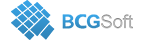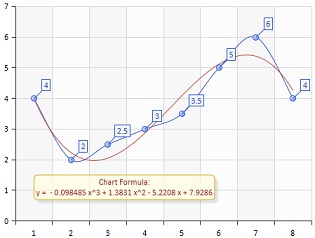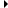BCGSuite for MFC
CBCGPChartTrendFormula

Detailed Description

The CBCGPChartTrendFormula class calculates coefficients for trend lines of different types. When a Trend Formula object is attached to a series, the series is drawn as a non-discrete curve on the diagram.

A Trend Formula object allows to specify only one input series. Then, depending on selected approximation type, it calculates coefficients using data points from the input series and generates screen positions for a non-discrete curve.

Currently the following trend lines are supported:

• Linear (y = a * x + b)
• Exponential (y = a * exp(b*x))
• Power (y = a * pow (x, b))
• Logarithmic (y = a * log(x) + b)
• Polynomial of order n = [2 - 6] (y = kn * x ^ n + kn-1 * x ^ (n - 1) + ... + k0)

The following picture illustrates a polynomial trend line of order 3:If you don't specify the input series, you can set your custom coefficients (AddCoefficient()) and display a curve calculated using the formulas above.

You can override CalculateTrendCoefficients() and OnCalculateYValue() methods to implement custom formulas.

The following code displays a polynomial trend line of order 3:

Copy
CBCGPChartVisualObject* pChart = m_wndChart.GetChart();
CBCGPChartSeries* pInputSeries = pChart->CreateSeries(_T("Input Series"));
// ... add data points to input series
CBCGPChartSeries* pSeriesResult = pChart->CreateSeries(_T("Trend Line"));
// set polynomial type
// set input series
formula.SetInputSeries(pInputSeries);
// set polynomial trend order
formula.SetPolynomialTrendOrder(3);
pSeriesResult->SetFormula(formula);

You can display a trend line on predefined range using SetTrendRange(). Call GetFormulaText() to retrieve a string with the formula text.

Note. After a formula object has been added to a series, you must use CBCGPChartSeries::GetFormula to obtain a pointer to the formula and modify it.

CBCGPChartBaseFormulaInheritance diagram for CBCGPChartTrendFormula:

Public Types

enum  TrendLineType

Public Member Functions

CBCGPChartTrendFormulaConstructs a CBCGPChartTrendFormula object.

GeneratePointsBuilds an array of screen positions of non-discrete curve representing a formula on a diagram.

GetCoefficientsReturns an array of coefficients.

GetFormulaTextReturns formula text.

GetPolynomialTrendOrderReturns polynomial trend order.

GetScreenPointsReturns screen positions of non-discrete curve representing a trend line.

GetTrendRangeReturns current trend line range.

GetTrendTypeReturns current trend line type.

IsNonDiscreteCurveOverridden. Always returns TRUE.

RemoveAllCoefficientsRemoves all coefficients.

SetCoefficientsSets an array of coefficients.

SetInputSeriesSets input series.

SetPolynomialTrendOrderSets polynomial trend line order.

SetTrendRangeSets trend line range.

SetTrendTypeSets new trend line type.Public Member Functions inherited from CBCGPChartBaseFormula
FindInputSeriesIndexFinds input series index.

GetInputSeriesAtReturns an input series located at the specified position.

GetInputSeriesCountReturns the number of input series.

GetLParamReturns user-defined data.

GetParentSeriesReturns a pointer to a parent series.

SetLParamSets user-defined data.

SetParentSeriesSets a parent series that represents a formula on the diagram.

Protected Member Functions

OnCalculateYValueCalled to calculate Y value for a formula y = f(x).